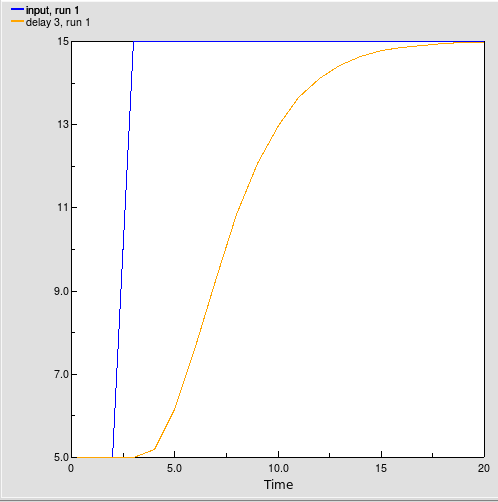# Built-in functions : delay1, delay3, delayn functions

delay1, delay3, delayn functions

delay1(input, duration [, initial])

delay3(input, duration [, initial])

delayn(input, duration, n [, initial])

Arguments:

input: the value to be delayed

duration: time by which to delay the input value

n (delayn only): order of the material delay (delayn only)

initial (optional): the value of the result when the function first applies

Result:

The delayed value of input.

The delay1, delay3 ​and delayn function calculates a first, third or nth-order material delay of input, using an exponential delay time of delay duration, and an optional initial value initial for the delay. delay3 does this by setting up a cascade of three first-order material delays, each with a delay duration of delay duration/3. Other versions of the function behave analogously. delay3 returns the value of the final delay in the cascade. If you do not specify an initial value initial, all functions assume the value to be the initial value of input.

The delay3 function will return the value of delay 3 in the structure and equations shown in the following figure:Compartment   comp1 :
Initial value = start_fill (real)
Compartment   comp2 :
Initial value = start_fill (real)
Compartment   comp3 :
Initial value = start_fill (real)
Flow   delay 1 :
delay 1 =         comp1*3/duration (1/day)
Flow   delay 2 :
delay 2 =         comp2*3/duration (1/day)
Flow   delay 3 :
delay 3 =         comp3*3/duration (1/day)
Flow   inflow :
inflow =         input (1/day)
Variable   start fill :

start fill =         initial*duration/3 (real)

Example:

Delay 3 = delay3(input, 5) where input = 5 + step(10,3) produces the pattern shown below: